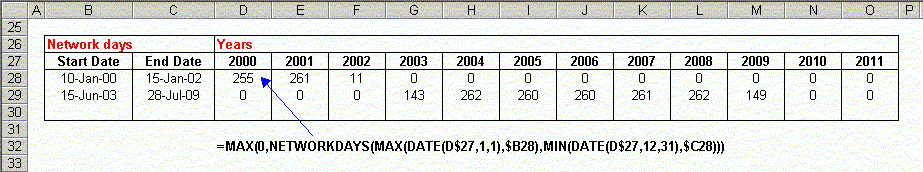Distributing Days Over Intervals

 Excel is frequently used as a platform to build scheduling applications.  In these sort of applications,  it may be useful to determine how the period between the start date and the end date are distributed across the intervening months or years.  For example, if a project starts on 12-February and ends on 28-June, how many days are used in each month?  And what about working days (Monday through Friday)?   Here, we'll look at a few formulas that will allow you do write these sorts of schedules.   NOTE:  Some of these formulas use the NETWORKDAYS function, which returns the number of working days between two dates.  This function is part of the Analysis Tool Pack, so you must have this installed in order to use these formulas.  For more information about installing the ATP, click here.   Distributing Working Days Across Months  You can distribute the number of working days between two  dates over the intervening months.  See the table below, for an example.This formula works essentially the same as the formula in the previous section, but uses subtraction instead of  NETWORKDAYS to count the number of days.  You can have more than the 12 months as shown in the example, and the first month does not need to be January.  For example if your Start Date is in June-2000, and your end date is in March-2003, you start with June-2000 in D5 and extend that our for 34 columns to AK5, which would contain March-2003.  Nothing in the formula requires 12 or fewer months, or that the dates be in the current year.  It will work properly for any number of months (up to 256, which is the limit of the number of columns in Excel).     Distributing Working Days Across Years  The previous sections explained how to distribute the days in an interval over a number of months.  You can also do something similar to distribute the working days between Start Date and End Date over years, instead of months.  In the example below, the interval between Start Date and End Date is distributed of the years 2000 to 2002 and 2003 and 2009.This formula works in a similar manner to the formula in the first section.  However, the year values in D5 to O5 are not Excel serial dates.  The actual value of the cell is the year number.  For example, D27 contains the number 1000.  The formula used to distribute the days over the years is =MAX(0,NETWORKDAYS(MAX(DATE(D\$27,1,1),\$B28),MIN(DATE(D\$27,12,31),\$C28)))This formula works for each year by first determining  the larger (later) of the Start Date (\$B28) and the first day of the year with the function MAX(DATE(D\$27,1,1),\$B28).  This will be the first day of the year for all years except the first.  Then, it determines the earlier (smaller) of the the End Date and the last day of the year with the function  MIN(DATE(D\$27,12,31),\$C28)). This will be the last day of the year for all years except the last. Next, it uses the NETWORKDAYS function  to determine the number of working days between the these two dates: NETWORKDAYS(MAX(DATE(D\$27,1,1),\$B28),MIN(DATE(D\$27,12,31),\$C28))Since NETWORKDAYS may return a negative number, we wrap the entire function in a MAX to return a zero if NETWORKDAYS returns a negative number: =MAX(0,NETWORKDAYS(MAX(DATE(D\$27,1,1),\$B28),MIN(DATE(D\$27,12,31),\$C28))) Distributing All Days Across Years  Finally, you may want to distribute all days, not just the working days, across a number of years.  The formula to do this is =MAX(0,(MIN(\$C39,DATE(D\$38,12,31))-MAX(\$B39,DATE(D\$38,1,1))+1))as shown in the following example.This formula works essentially the same as the formula in the previous section, but uses subtraction instead of  NETWORKDAYS to count the number of days.  You can download a file illustrating these formulas (10KB zipped).

 Other Date And Time Related Procedures are described on the following pages. Adding Months And Years The DATEDIF Function Date Intervals Dates And Times Date And Time Entry Date And Time Arithmetic Distributing Dates Over Intervals Holidays Julian Dates Latitude And Longitude  Overtime Hours And Timesheets VBA Procedures For Dates And Times Week Numbers Worksheet Functions For Dates And Times See the Dates And Times Topic Index For Information